Mobile QR Code1. (Department of System Semiconductor Engineering, Sangmyung University, 31 Sangmyungdae-gil, Dongnam-gu, Cheonan, Chungnam, 31066, Korea)

Quantum-mechanical correction, 1/f gate leakage current noise, 1/f drain current noise, random telegraph noise, nanoscale MOSFETs

## I. INTRODUCTION

With the introduction of MOS devices aggressively scaled to deep submicron channel length, an ultrathin gate oxide and high doping concentration are required to increase the drive current and to minimize undesirable short channel effects. These design rules result in strong transverse electric fields at the Si-SiO2 interface even near the threshold of inversion. This gives rise to significant band bending of the energy bands, leading to the confinement of electron motion in the direction perpendicular to the interface. In these conditions, electrons in the inversion layer form actually a quasi twodimensional electron gas (2-DEG) with quantized energy levels within the channel. These quantum mechanical (QM) effects have significant impact on the performance of nanoscale MOSFETs and thus the classical models are inadequate for state-of-the-art MOS structures[2-4].

The quantum nature of electrons in the inversion layer is typically studied by solving Schrödinger’s and Poisson’s equations self-consistently, and also considering the simplified models used in circuit simulators. The enhancement in the centroid and the finite thickness of the inversion layer are one of the main QM effects on MOSFET behavior. This reduces the inversion layer charge density and the total gate oxide capacitance[8,9], compared to classical models without QM effects, and causes the threshold voltage shift effect [10,11].

For a compact circuit simulation model of direct tunneling of electrons form nMOS inversion layer, a variational approximation is employed to calculate the surface potential and the energy of the first quantized level in the inversion layer without the requirement for iterative solution, and an efficient model is demonstrated by using both QM calculations for the substrate and a modified WKB approximation for the transmission probability. It is shown from the quantum charge sheet model that QM effects on the drain current are expected to be due to QM effects on gradients in both the inversion charge and in the surface potential .

Besides QM effects on the static characteristics, QM effects have been observed in the trap and noise behavior [4,[15-19]. For heavily doped MOSFETs, extracted interface trap capture cross sections are found to be an order of magnitude larger than values from the classical theory. In order to investigate QM effects on the capture and emission times of random telegraph signal (RTS) noise, the interaction between an oxide trap and an electron in the sub-bands of the inversion layer is theoretically modeled and analyzed in a Si-SiO2 interface [15,16,18]. Regarding the 1/f noise models of drain current, numerical models are employed to account for QM impact on the extracted value of the oxide trap density. However, there are no analytical 1/f and thermal noise models, which incorporate QM effects and are applicable to circuit simulations.

In this paper, a comprehensive charge-based compact model is newly formulated to quantify QM effects on both the gate and drain current noise in nanoscale MOSFETs. The modeling approach uses calculation of surface potential $\psi_{s}$ to capture the physics of quantum transport. An emphasis on surface-potential-based parameters of noise models is laid to make the models to accurately predict the noise performance over all operation regimes of nanoscale MOSFETs, as well as at low and high frequencies. Furthermore, with the help of an accurate and generally applicable compact noise model, we can implement the compact noise models into circuit simulator format. Section II describes the quantization effects on the inversion charge parameters. In Section III, on the basis of the quantum correction model of the inversion layer charge, a gate leakage current and 1/f noise model are formulated. Section IV gives the analytical expressions for the drain current and noise model accounting for quantum and short channel effects. The comparison between the classical and quantum models is shown in Section V. Finally, Section VI presents some conclusions.

## II. QUANTUM CORRECTION FOR COMPACT MODELING

### 1. Surface Potential and Threshold Voltage Model

The high channel doping levels and large transverse electric fields result in the confinement of electron motion in the x direction perpendicular to the interface, so that the conduction band within the channel is split into discrete sub-bands. Based on the effective mass approximation and a parabolic band structure, the envelope wave function $\zeta_{i j}$ for the ith valley and jth sub-band satisfies 1-D Schrödinger’s equation. Due to the nonzero value of the quantized charge centroid, the surface potential $\psi_{s}$ determined by quantum effects is greater than predicted by the classical model. The enhancement of the surface potential is given by the average centroid position from the interface, $x_{n}^{Q M}$ and the total electron charge density, $Q_{n}$[8,12,14,20-22].

##### (1)
$\Delta \psi_{s}^{QM}=\frac{Q_{n}^{QM}\left(\psi_{s}\right) \cdot x_{n}^{QM}\left(\psi_{s}\right)}{\varepsilon_{s i}}$
(1)

with

##### (2a)
$x_{n}^{QM}\left(\psi_{s}\right)=\frac{\sum_{i j} N_{i j} x_{i j}}{N_{n}^{Q M}\left(\psi_{s}\right)}$, $x_{i j}=\frac{2 E_{i j}}{3 q F_{s}\left(\psi_{s}\right)}$

##### (2b)
$Q_{n}^{Q M}\left(\psi_{s}\right)=q N_{n}^{Q M}\left(\psi_{s}\right)=\sum_{i j} q N_{i j} =\frac{q k T}{\pi \hbar^{2}} \sum_{i j} g_{i} m_{d i} \ln \left[1+\exp \left(\frac{E_{f}-E_{i j}}{k T}\right)\right]$

##### (2c)
$E_{f}-E_{i j}=q \psi_{s}-\frac{E_{g}}{2}-q \phi_{f}-E_{i j}$

##### (2d)
$E_{i j}=\left(\frac{\hbar^{2}}{2 m_{x i}}\right)^{1 / 3}\left[\frac{3}{2} q \pi F_{s}\left(\psi_{s}\right)\left(j+\frac{3}{4}\right)\right]^{2 / 3}$ for $j=0,1,2, \cdots$

where $\mathcal{E}_{S i}$ is the silicon dielectric constant, $q$ is the electron charge, $\hbar$ is the reduced Planck constant, $k$ is the Boltzmann’s constant, $T$ is the temperature, $g_{i}$ is the degeneracy of the energy sub-band ($g_{1}$=2, $g_{2}$=4), $m_{di}$ is the density-of-states effective mass ($m_{d1}$=$m_{x2}, m_{di2}= \left(m_{x 1} m_{x 2}\right)^{1 / 2}$), $m_{xi}$ is the effective mass perpendicular to the surface ($m_{x 1}$ = 0.916 $m_{0}$, $m_{x 2}$ = 0.19 $m_{0}$), $E _{ij}$ is the energy state of the $i$th valley and $j$th sub-band, $N _{ij}$ is the sheet charge density, $E _{f}$ is the Fermi energy level, $\phi_{f}=(k T / q) \ln \left(N_{s u b} / n_{i}\right)$ is the Fermi potential, $E _{g}$ is the energy bandgap, and $F _{s}$ is the surface electric field.

Due to the quantum-mechanical behavior of inversion layer electrons, more band bending is required to achieve the same inversion charge as the classical value, resulting in the increase in the threshold voltage. The threshold voltage $V _{T}$ can be easily calculated by including an additional quantum shift term as follows[11,22]

##### (3)
$V_{T}^{Q M}=V_{T}+\Delta V_{T}^{Q M}$

with

##### (4)
$\Delta V_{T}^{o M}=\Delta\left.\psi_{s}^{Q M}\right|_{\psi_{s}=2 \varphi_{f}} \cdot\left(1+\frac{1}{2 C_{o x}} \sqrt{\frac{\varepsilon_{s i} q N_{s i b}}{\varphi_{f}}}\right)$

where $N _{sub}$ is the substrate doping density, $n _{i}$ is the intrinsic carrier density, and $C _{ox}$ is the oxide capacitance per unit area.

For the polysilicon-SiO2-p-Si MOS structure, the relationship between the gate-to-source voltage $V _{gs}$ and the surface potential $\psi_{s}$ is described by the quantized charge density[8,12]

##### (5)
$V_{g s}=V_{F B}+\psi_{s}^{QM}+V_{p o l y}+\frac{q N_{n}^{QM}\left(\psi_{s}^{O M}\right)+Q_{d}\left(\psi_{s}^{QM}\right)}{C_{o x}}+\frac{Q_{i t}}{C_{o x}}$

with

##### (6)
$V_{p o l y}=\frac{q}{2 m}\left[\sqrt{1+4 m\left(V_{g s}-V_{F B}-\psi_{s}\right)}-1\right]$, $m=\frac{\varepsilon_{o x}^{2}}{2 q \varepsilon_{s i} N_{p o l y} t_{o x}^{2}}$

where $V_{F B}$ is the flat-band voltage, $V_{\text {poly}}$ is the polysilicon voltage drop, $N_{\text {poly}}$ is the polysilicon doping concentration, $\varepsilon_{ox}$ is the oxide dielectric constant, $t_{ox}$ is the oxide thickness, the trapped charge in the oxide, $\mathcal{Q}_{i t}$, is included to account for the trapping-detrapping effects at the Si-SiO2 interface, and $\mathcal{Q}_{d}$ is the depletion charge density per unit area.

### 2. Inversion Charge and Capacitance Model

As the channel length and oxide thickness continue to be scaled to nanometer range in current CMOS devices, the quantization effects should be taken into account for an accurate inversion charge model. By using Eqs. ((1), (5)), an expression for the inversion layer charge is obtained as

where $N _{sub}$ is the substrate doping density, $n _{i}$ is the intrinsic carrier density, and $C _{ox}$ is the oxide capacitance per unit area.

For the polysilicon-SiO2-p-Si MOS structure, the relationship between the gate-to-source voltage $V _{gs}$ and the surface potential $\psi_{s}$ is described by the quantized charge density[8,12]

##### (6b)
$Q_{n 0}^{Q M}\left(\psi_{s}^{Q M}\right)=C_{o x}^{Q M}\left(V_{g s}-V_{F B}-\psi_{s}^{QM}-\Delta \psi_{s}^{QM}-V_{p o y}-\frac{Q_{d}+Q_{i t}}{C_{o x}}\right) =C_{o x}^{Q M}\left(V_{g s}-V_{T}^{Q M}\right)$

with

##### (7)
$C_{o x}^{Q M}=\frac{\varepsilon_{o x}}{t_{o x}^{Q M}}=\frac{\varepsilon_{o x}}{t_{o x}+\frac{\varepsilon_{o x}}{\varepsilon_{s i}} x_{n}^{Q M}}$

where the denominator $t_{o x}^{Q M}$ of Eq. (7) is termed the electrical oxide thickness. Including the parasitic source and drain resistance $R_{s, d}$ and incorporating the drain-tosource voltage $V_{ds}$, the channel charge density is expressed by

##### (8)
$Q_{n}^{QM}(y)=C_{o x}^{Q M} \cdot\left(V_{g s}-I_{d} R_{s, d}-V_{T}^{QM}-2 k_{b u l k} V_{y}(y)\right)$

where $I _{d}$ is the drain current, $V _{y} (y)$ is the channel potential at any given point y along the channel and $k_{bulk}$ is the bulk charge parameter.

Since the quantum effects lead to an increase in the inversion layer thickness, the inversion capacitance decreases and thus, the total gate capacitance is reduced. The inversion charge capacitance is given by [7,22]

##### (9)
$\frac{1}{C_{n}^{Q M}}=\frac{1}{C_{n}}+\frac{X^{\varrho M}}{\varepsilon_{s i}}$

with

##### (10a)
$X^{QM }=\frac{\int x \cdot n(x) d x}{\int n(x) d x}=\frac{5.047+0.771 E_{e f f}}{0.113+E_{e f f}}$

##### (10b)
$E_{e f f}=\frac{1}{2 t_{o x}}\left[n_{s w} v_{t h} \ln \left(1+\exp \left(\frac{V_{g s}-V_{p o y}-V_{T}}{n_{s w} v_{t h}}\right)\right)+2\left(V_{T}-V_{F B}-2 \varphi_{f}\right)\right]$

##### (10c)
$n_{s w}=1+\sqrt{\frac{\varepsilon_{s i} q N_{s u b}}{4 \varphi_{f} C_{o x}^{2}}}$

where Cn is the classical inversion capacitance, $X^{Q M}$ is the inversion layer thickness, $E _{eff}$ is the effective electric field in inversion layer in MV/cm, and $n _{sw}$ is the subthreshold swing parameter. For very high polysilicon doping levels ($N _{poly}$ > 1019 cm-3), no quantum behavior has to be considered to determine the surface potential at the polysilicon-SiO2 interface, because all the subbands are squeezed out of the potential well. According to the classical theory, the polysilicon capacitance is calculated by differentiating the polysilicon charge density $Q _{poly}$ as follows

##### (11)
$C_{p o l y}=\frac{\sqrt{2 \varepsilon_{s i} q N_{p o l y}}\left[\exp \left(\frac{V_{p o l y}}{v_{t h}}\right)-1\right]}{\sqrt{v_{t h} \exp \left(\frac{V_{p o l y}}{v_{t h}}\right)+V_{p o l y}-v_{t h}}}$

## III. QUANTUM EFFECTS ON GATE LEAKAGE CURRENT AND NOISE MODEL

### 1. Gate Leakage Current Model

In the ultrathin gate oxide regime ( < 3 nm), the classical models without including quantum effects of the inversion layer overestimate the value of the gate leakage current and noise, because the peak charge density is situated at a distance (~1 nm) away from the Si-SiO2 interface. This leads to an enhancement in the oxide thickness and a reduction in the oxide voltage. Therefore, the quantum effects need to be taken into account by using the correction for the oxide thickness and the oxide voltage,

##### (12)
$V_{o x}^{Q M}=V_{g s}-V_{F B}-\psi_{s}^{Q M}-V_{p o l y}-\frac{Q_{i t}}{C_{o x}}$

The BSIM4 gate tunneling current model can predict all the significant direct tunneling current components which are electron tunneling from the conduction band in the substrate to the gate, electron tunneling from the valence band in the substrate to the gate, and hole tunneling from the valence band in the gate to the substrate. Taking into account quantum effects of the oxide thickness and the oxide voltage, the tunneling current model becomes[12,23]

##### (13)
$J_{D T, B SI M}^{QM}=\frac{q^{3}}{8 \pi h \varphi_{b} \varepsilon_{o x}} \cdot C^{Q M}\left(t_{o x}^{O M}, V_{o x}^{Q M}\right) \cdot \exp \left[-\frac{8 \pi \sqrt{2 m_{o x}} \varphi_{b}^{3 / 2} t_{ox}^{QM}\left[1-\left(1-\left|V_{o x}^{QM}\right| / \varphi_{b}\right)^{3 / 2}\right]}{3 h q\left|V_{o x}^{QM}\right|}\right]$

with

##### (14a)
$C^{Qm}\left(t_{o x}^{Q M}, V_{o x}^{Q M}\right)= \exp \left[\frac{20}{\varphi_{b}}\left(\frac{\left|V_{o x}^{QM}\right|-\varphi_{b}}{\varphi_{b o}}+1\right)^{\lambda} \cdot\left(1-\frac{\left|V_{o x}^{QM}\right|}{\varphi_{b}}\right)\right] \cdot\left(\frac{V_{g s}}{t_{o x}^{Q M}}\right) \cdot N^{QM}$

##### (14b)
$N^{Q M}=\frac{\varepsilon_{o x}}{t_{o x}^{Q M}}\left\{n_{s w} \ln \left[1+\exp \left(\frac{V_{g s}-V_{p o l y}}{n_{s w}}\right)\right]+v_{t h} \ln \left[1+\exp \left(-\frac{V_{g s}-V_{f b}}{v_{t h}}\right)\right]\right\}$

where $h$ is the Planck constant, $m_{ox}$ is the electron effective mass of the conduction band in the oxide, $\lambda$ is a fitting parameter for three current components, $\phi_{b o}$ is the Si-SiO2 barrier height (3.1 eV for electrons and 4.5 eV for holes), and $\phi_{b}$ is the tunneling barrier height.

### 2. Gate Current Noise Model

One possible mechanism for 1$/ f^{\gamma}$ noise of the gate leakage current is for traps to affect locally the barrier height or shape due to thermal noise of the frequencydependent conductance of the oxide slow traps . This fluctuation in turn modulates the tunneling transmission, and causes fluctuations in the tunneling current. The quantum effects for the spectral noise density are accounted for by the correction of the oxide voltage $V_{ox}$ and the electron sub-band energy $E_{ij}$, and are described by 

##### (15)
$\left.\frac{S_{I_{g}}^{1 / f}(f)}{I_{g}^{2}}\right|^{\text { QM }}= \frac{\frac{G_{p}^{QM}(f)}{2 \pi f}}{\left[\frac{G_{p}^{\text { QM }}(f)}{2 \pi f}\right]^{2}+\left[C_{o x}^{\text { QM }}+C_{p}^{\text { QM }}(f)\right]^{2}} \cdot \frac{1}{f} \cdot A^{QM}\left(V_{o x}^{QM}\right) \cdot \sum_{i j} B_{i j}^{O M}\left(V_{o x}^{QM}, E_{i j}\right)$

with

##### (16a)
$A^{Q M}\left(V_{o x}^{Q M}\right)=\frac{16 m_{o x} k T q^{2}}{9 \pi \hbar^{2} A_{g}\left(t_{o x}^{Q M}\right)^{2}\left(X_{2}^{Q M}\right)^{4}}$

##### (16b)
$B_{i j}^{Q M}\left(V_{o x}^{Q M}, E_{i j}\right)=\sqrt{X_{1, i j}^{Q M}\left(X_{1, i j}^{Q M}-q V_{o x}^{Q M}\right.} )\left[8 X_{1, i j}^{Q M}\left(X_{1, i j}^{Q M}-q V_{o x}^{Q M}\right)-3 q^{2}\left(V_{o x}^{Q M}\right)^{2}\right]$

$-2\left[2\left(X_{1, i j}^{Q M}\right)^{2}\left(2 X_{1, i j}^{Q M}-3 q V_{o x}^{Q M}\right)+q^{3}\left(V_{o x}^{Q M}\right)^{3}\right]$

##### (16c)
$X_{1, i j}^{Q M}=\varphi_{b}+E_{f s}-E_{i j}+\frac{m_{\perp}}{m_{o x}} E_{\perp}$, $X_{2}^{Q M}=q \frac{V_{o x}^{Q M}}{t_{o x}^{Q M}}$

where $m_{\perp}$ is the transversal electron effective mass, $E_{\perp}$ is the transversal energy component, $A_{g}$ is the gate tunneling area, $G_{p}^{Q M}(f)$ and $C_{p}^{Q M}(f)$ are the frequencydependent parallel conductance and capacitance of oxide traps, respectively. Assuming that the oxide trap density is spatially distributed over a physical distance d into the oxide, the electrical trap distance $d^{Q M}$ becomes $d+\left(\varepsilon_{o x} / \varepsilon_{s i}\right) x_{n}^{Q M}$ in the quantum approach, leading to

##### (17)
$\frac{G_{p}^{QM}(f)}{2 \pi f}=\left.\frac{G_{p}(f)}{2 \pi f}\right|_{d \rightarrow d^{QM}}$, $C_{p}^{Q M}(f)=C_{p}\left.(f)\right|_{d \rightarrow d^{Q M}}$

## IV. QUANTUM EFFECTS ON DRAIN CURRENT AND NOISE MODEL

### 1. Mobility and Drain Current Model

As the gate oxide thickness and the substrate doping level are thinner and higher, the normal electric field increases significantly and the influence of the quantized inversion layer become important. A correct and simple model to account for these effects is required to predict the mobility and $I-V$ characteristics of nanoscale MOSFETs. The quadratic mobility degradation factor is necessary for an effective mobility model of ultrathin gate oxide nMOSFETs, and an analytical mobility model $\mu_{n, e f f}^{Q M}$ is given by

##### (18)
$\mu_{n, e f f}^{QM}= \frac{\mu_{n 0}}{1+\alpha_{1}^{Q M}\left(\frac{V_{g s}-V_{T}^{Q M}-k_{b u l k} V_{d s}}{t_{o x}^{Q M}}\right)+\alpha_{2}^{Q M}\left(\frac{V_{g s}-V_{T}^{Q M}-k_{b u l k} V_{d s}}{t_{o x}^{Q M}}\right)^{2}}$

where $\mu_{n 0}$ is the low-field electron mobility, and $\alpha_{1}^{Q M}$ and $\alpha_{2}^{Q M}$ are the mobility degradation factor. Introducing an effective drain-to-source voltage $V_{d s, e f f}^{Q M}$, and considering the channel length shortening effects and parasitic resistance $R_{s,d}$, the drain current for shortchannel MOSFETs biased in the linear and saturation region is then obtained as [22,26]

##### (19)
$I_{d, t o t a l}^{Q M}=\frac{W}{L} \mu_{n, e f f}^{Q M} C_{o x}^{Q M} \frac{\left(V_{g s}-V_{T}^{Q M}-k_{b u l k} V_{d s, e f f}^{Q M}\right) \cdot V_{d s, e f f}^{Q M}}{\left(1+\frac{V_{d s, e f f}^{Q M}}{E_{c} L}\right)+\frac{W}{L} \mu_{n, e f f}^{Q M} C_{o x}^{Q M} R_{s, d} V_{d s, e f f}^{Q M}-\frac{L_{s a t}}{L}}$

with

##### (20a)
$V_{d s, e f f^{r}}^{Q M}=\frac{1}{2}\left(V_{d s}+(1+\Delta) V_{d s a t}^{Q M}\right)-\sqrt{\frac{1}{4}\left(V_{d s}+(1+\Delta) V_{d s a t}^{Q M}\right)^{2}-V_{d s} \cdot V_{d s a t}^{Q M}}$

##### (20b)
$V_{d s a t}^{Q M}=\left.V_{d s a t}\right|_{C_{o x} \rightarrow C_{\partial x}^{Q M}, V_{T} \rightarrow V_{T}^{Q M}}$

where $W$ is the channel width, $L$ is the channel length, $E_{c}$ is the lateral critical electric field at which carriers travel with the saturated velocity $v_{sat}$ near the drain section of the length $L_{sat}$, and $\Delta$ is a small quantity to adjust the smoothness at the transition region near $V_{dsat}$ .

### 2. RTS (Random Telegraph Signal) Noise Model

If we consider a section of channel with width $W$ and length $y$, fluctuations in the trapped oxide charge will induce correlated fluctuations in the channel carrier number and mobility. The resulting fractional change in the local drain current can be represented as [15,27]

##### (21)
$\frac{\Delta I_{d}}{I_{d}}=-\left(\frac{1}{\Delta Q_{n}} \cdot \frac{\delta \Delta Q_{n}}{\delta \Delta Q_{T}} \pm \frac{1}{\mu_{n, e f f}} \cdot \frac{\delta \mu_{n e f f}}{\delta \Delta Q_{T}}\right) \cdot \delta \Delta Q_{T}$

where $\Delta Q_{n}=Q_{n} W \Delta y$ , $\Delta Q_{T}=Q_{T} W \Delta y$ , and $Q_{n}$ and $Q_{T}$ are total charge of channel carriers and occupied traps per unit area. The sign of the mobility term is chosen either positive for acceptor-like traps or negative for donor-like traps. The ratio $R_{0}=-\delta \Delta Q_{n} / \delta \Delta Q_{T}$ in Eq. (21) describes the efficiency of the drain current modulation corresponding to a variation of the trapped charge. Considering the effects of the quantization and polysilicon depletion, the coefficient $R_{0}^{Q M}$ depends on the surface potential through charge capacitance and is given by 

##### (22)
$R_{0}^{Q M}=\frac{C_{n}^{Q M}}{C_{o x}^{Q M}+C_{n}^{Q M}+C_{d}^{Q M}+C_{p o l y}+C_{i t}}=\frac{Q_{n}^{Q M}}{Q_{n}^{Q M}+Q_{n}^{Q M^{*}}}$

with

##### (23)
$C_{n}^{Q M} \approx \frac{q}{k T} Q_{n}^{Q M}$, $Q_{n}^{Q M^{*}}=\frac{k T}{q}\left(C_{o x}^{Q M}+C_{d}^{Q M}+C_{p o l y}+C_{i t}\right)$

where $C_{it}$ is the interface trap capacitance. On the basis of Matthiessen’s rule, we can readily find that

##### (24)
$\delta \mu_{n, e f f}=\mu_{n, e f f}^{2} S^{Q M}\left(x_{t}^{Q M}, Q_{n}^{Q M}\right) \cdot \frac{\delta \Delta Q_{T}}{q W \Delta y}$

where $S^{Q M}\left(x_{t}^{Q M}, Q_{n}^{Q M}\right)$ is a scattering rate which is a function of the distance $x_{t}^{Q M}\left(=x_{t}+\left(\varepsilon_{o x} / \varepsilon_{s i}\right) x_{n}^{Q M}\right)$ of the trapping state and of the carrier charge density $Q_{n}^{QM}$ , and is strongly affected by channel quantization. In order to properly account for these effects in ultrathin oxide MOSFETs, we employ an empirical model for the scattering coefficient $S^{Q M}\left(x_{t}^{Q M}, Q_{n}^{Q M}\right)$ in linear approximation as [28,29]

##### (25)
$S^{Q M}\left(x_{t}^{Q M}, Q_{n}^{Q M}\right)=S_{1}-S_{2} \ln \left(\frac{Q_{n}^{Q M}}{q}\right)+S_{3} \ln \left(\frac{r_{\max }}{x_{t}^{Q M}}\right) \approx \frac{1}{S_{0} \sqrt{x_{t}^{Q M} / r_{\max }^{Q M}} \cdot \sqrt{Q_{n}^{Q M}}}$

where $r_{\max }^{Q M} \approx\left(\varepsilon_{o x}+\varepsilon_{s i}\right) /\left(C_{o x}^{Q M}+C_{d}^{Q M}+C_{i t}\right)$ is the maximum distance from the scattering charge for which the effect of image charges can be neglected and $S_{0}$ is the scattering constant in cm/C1/2Vs. Assuming that the carrier distribution is uniform along the channel, the RTS amplitude noise becomes 

##### (26)
$\frac{\Delta I_{d}}{I_{d}}=-\frac{1}{W L} \left(q \frac{R_{0}^{Q M}}{Q_{n}^{Q M}} \cdot \pm S^{Q M}\left(x_{t}^{Q M}, Q_{n}^{Q M}\right) \cdot \mu_{n, e f f}^{Q M} \right)$

### 3. Low and High Frequency Noise Model

According to the number fluctuation theory, the total power spectral density of the drain current noise at low frequencies is obtained by calculating the fluctuations in the number of occupied traps and performing the integration over the channel length. Assuming that the oxide trap density $N_{t} (E)$ is uniformly distributed over the space into the oxide and the trapping time constant is an exponentially increasing function of the trap distance $x_{t}$, the total $1/f$ noise power of the drain current in the linear region ($V_{d} \leq V_{d s a t}$) is given by 

##### (27)
$S_{I_{d}}^{1 / f}\left.(f)\right|_{L i n} ^{Q M}= \frac{k T q^{2} I_{d}^{Q M} \mu_{n, \epsilon f f}^{Q M}}{2 k_{b u l k} \gamma_{n m} f L^{2} C_{o x}^{Q M}} \left[A^{Q M} \ln \left(\frac{Q_{n 0}^{Q M}+Q_{n}^{Q M^{*}}}{Q_{n L}^{Q M}+Q_{n}^{Q M^{*}}}\right)+\frac{B^{Q M}}{q}\left(Q_{n 0}^{Q M}-Q_{n L}^{Q M}\right)+\frac{C^{Q M}}{2 q^{2}}\left(\left(Q_{n 0}^{Q M}\right)^{2}-\left(Q_{n L}^{Q m}\right)^{2}\right)\right]$

with

##### (28a)
$A^{Q M}=N_{t}\left(E_{f n}\right)$, $B^{Q M}=\frac{2 S^{Q M} \mu_{n, e f f}^{Q M}}{R_{0}^{Q M}} N_{t}\left(E_{f n}\right)$, $C^{Q M}=\left(\frac{S^{Q M} \mu_{n, e f f}^{Q M}}{R_{0}^{Q M}}\right)^{2} N_{t}\left(E_{f n}\right)$

##### (28b)
$Q_{n 0}^{Q M}=C_{\alpha x}^{Q M}\left(V_{g s}-V_{T}^{Q M}\right)$, $Q_{n L}^{Q M}=C_{o x}^{Q M}\left(V_{g s}-V_{T}^{Q M}-2 k_{b u l k} V_{d s}\right)$

where $\gamma_{t u n} \approx 10^{8} \mathrm{cm}^{-1}$ is the exponential factor of tunneling process, $N_{t}(E_{fn})$ is the oxide trap density around the electron quasi-Fermi level $E_{fn}$, and $Q_{n 0}^{Q M}$ and $Q_{n L}^{Q M}$ are carrier charge densities for $R_{s,d} = 0$ at the source and drain ends of the channel, respectively. In the saturation region, the total $1/f$ noise power is obtained by including the noise contribution arising from the pinchoff region $L_s{at}$, and is represented as 

##### (29)
$S_{I_{d}}^{1 / f}\left.(f)\right|_{S a t} ^{Q M}= S_{I_{d}}^{1 / f}\left.(f)\right|_{L i n} ^{Q M}+L_{s a t} \frac{k T\left(I_{d}^{Q M}\right)^{2}}{\gamma_{\text {tun}} f W L^{2}} \frac{q^{2} A^{Q M}+q B^{Q M} Q_{n L}^{Q M}+C^{Q M}\left(Q_{n L}^{Q M}\right)^{2}}{\left(Q_{n L}^{Q M}+Q_{n}^{Q M^{*}}\right)^{2}}$

with

##### (30)
$Q_{n 0}^{Q M}=C_{o x}^{Q M}\left(V_{g s}-V_{T}^{Q M}\right)$, $Q_{n L}^{Q M}=C_{o x}^{Q M}\left(V_{g s}-V_{T}^{Q M}-2 k_{b u l k} V_{d s a t}\right)$

It has been shown that the high frequency noise originates from thermal fluctuation in the channel and is expresses as [30,35]

##### (31)
$S_{I_{d}}^{T h e r m a l}=4 k T \cdot \frac{\mu}{L^{2}} \cdot Q_{n}\left(V_{g s}, V_{d s}\right)$

where $Q_{n}$ is the total inversion layer charge density. For the thermal noise modeling of short channel devices, the total inversion charge density is derived by considering the velocity saturation of carriers due to the high lateral electric field, and is turned out to be 

##### (32)
$Q_{n}^{Q M}\left(V_{g s}, V_{d s}\right)=\frac{W^{2} \mu_{e f f}^{Q M}\left(C_{o x}^{Q M}\right)^{2}}{I_{d}^{g M}} \left[\left(V_{g s}-V_{T}^{QM}\right)^{2} V_{d s}-2 k_{b u l k}\left(V_{g s}-V_{T}^{Q M}\right) V_{d s}^{2}+\frac{4}{3} k_{b u l k}^{2} V_{d s}^{3}\right]$

$-\frac{W C_{o x}^{QM}}{E_{c}} \left[\left(V_{g s}-V_{T}^{Q M}\right) V_{d s}-k_{b u l k} V_{d s}^{2}\right]$

Substituting Eq. (32) into Eq. (31), the thermal noise spectral densities of drain current in the linear and saturation region are given by

##### (33a)
$\left.S_{I_{d}}^{\text {Thermal}}\right|_{L i n} ^{Q M}= 4 k T \cdot \frac{\mu_{n, e f f}^{Q M}}{L^{2}} \cdot Q_{n}^{Q M}\left(V_{g s,} V_{d s}\right)$

##### (34b)
$\left.S_{I_{d}}^{T h e r m a l}\right|_{S a t} ^{Q M}=4 k T \cdot \frac{\mu_{n, e f f}^{Q M}}{\left(L-L_{s a t}\right)^{2}} \cdot Q_{n}^{Q M}\left(V_{g s} V_{d s a t}\right)$

## V. RESULTS AND DISCUSSION

For the purpose of developing a model applicable to circuit simulation, it is very important to extend classical simulators to include QM effects with computational efficiency. For the quantized energy levels of inversion layers, a simple and approximate model can be obtained by considering the dominant contribution of carriers in the lowest energy sub-bands $E_{10}$. Fig. 1(a) and Fig. 1(b) show the inversion charge density and capacitance as a function of the gate-source voltage according to the classical and QM models, respectively. The deviation between the classical and QM models for the inversion charge density increases significantly for higher doping concentration, and for the inversion layer capacitance, the results coincide in weak inversion and deviate in strong inversion. For the feasibility of QM model, results reported by other researchers are also demonstrated in Fig. 1(a). It is clearly shown that QM model are satisfactorily coincident with previous results.

Fig. 1. Quantum effects on (a) the inversion charge density $Q_{n0}$ versus the gate-source voltage $V_{gs}$, (b) the inversion layer capacitance $C_{n}$ versus $V_{gs}$ for $N_{sub}$ = 3×1017 cm-3 and 3×1018 cm-3. ($t_{ox}$ = 2.5 nm, $V_{FB}$ = -0.9 V, $E_{ij}$ = $E_{10}$, $N_{it}$ = 2×1011 cm-2, $N_{poly}$ = 2×1020 cm-3, $T$ = 300 K).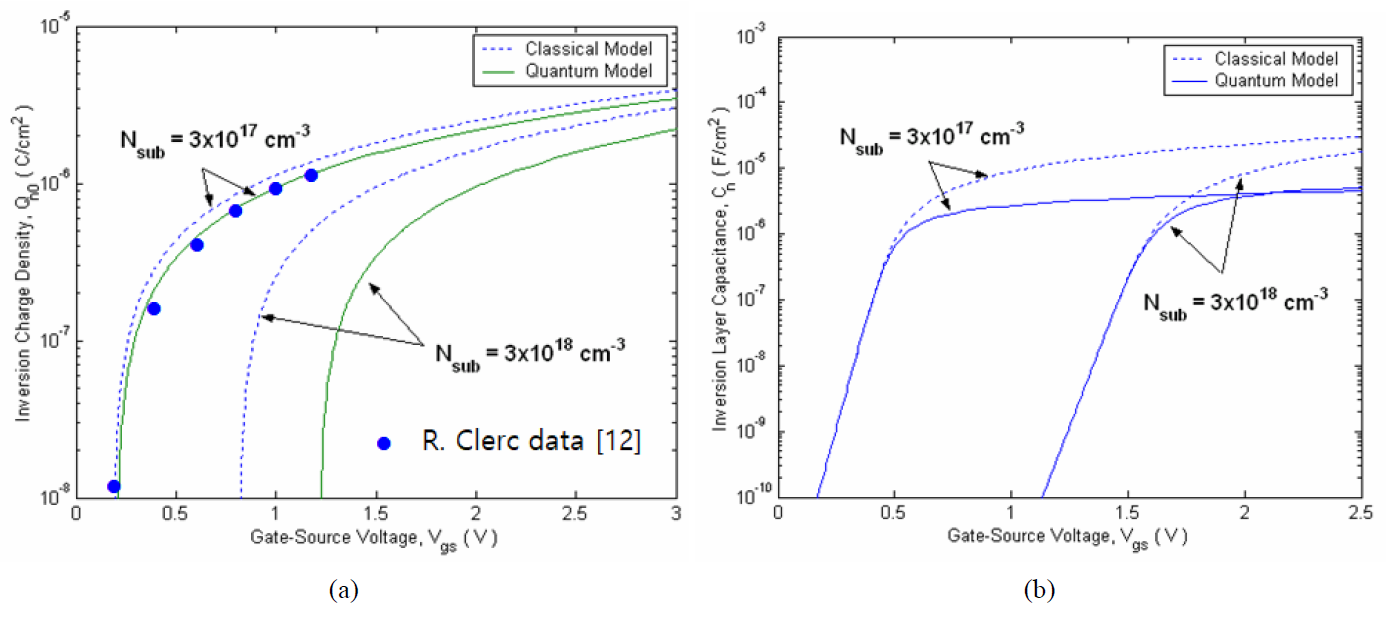Fig. 2(a) illustrates the gate leakage current noise characteristics as a function of drain-source voltage for two channel lengths, verifying that the classical model have good agreement with measurements for $t_{ox}$=2.5 nm . For thinner oxide MOS structure, however. the oxide thickness shift due to QM effects has a greater impact on the gate tunneling current than the oxide voltage shift due to QM effects. By considering the QM effects on the oxide voltage and the oxide thickness, the gate leakage tunneling current and noise model can be formulated. The oxide voltage due to QM effects is calculated using the additional surface potential to achieve the same inversion charge as the classical value. As illustrated in Fig. 2(b), the deviation between the classical and QM model increases with decreasing oxide thickness. The use of the physical oxide thickness ignoring QM effects leads to erroneous results in the calculation of the gate tunneling current noise. This observation is in agreement with the results reported in , showing similar values of normalized gate current noise for the oxide thickness ~1.5 nm.

Fig. 2. (a) Comparison of the simulation results of classical model and measured data of the gate current noise as a function of drain-source voltage $V_{ds}$ ($t_{ox}$ = 2.5 nm), (b) quantum effect on the normalized $1/f$ noise of gate leakage current $S_{Ig}$/$I_{g}$ versus the gate-source voltage $V_{gs}$ for two oxide thickness ($N_{sub}$ = 3×1017 cm-3, $V_{FB}$ = -0.9 V, $E_{ij}$ = $E_{10}$, $N_{it}$ = 2×1011 cm-2, $N_{poly}$ = 2×1020 cm-3, $T$ = 300 K).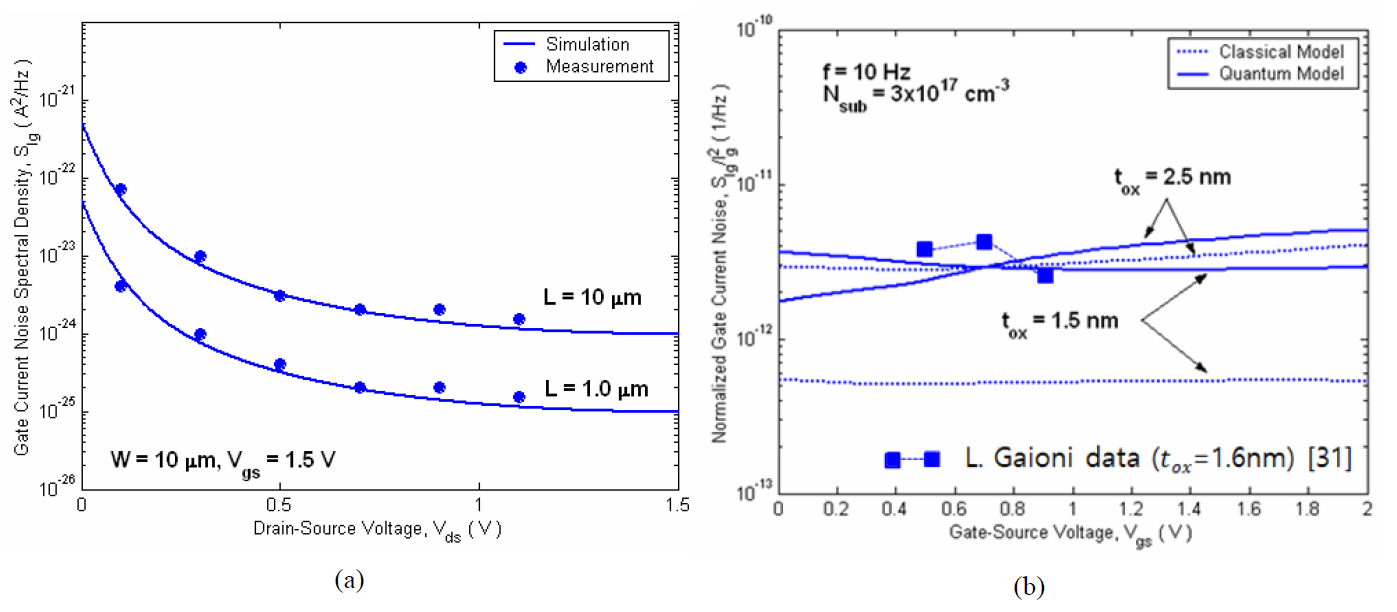On the basis of the inversion charge model including QM effects, the drain current and noise model is evaluated for ultrathin oxide MOS device ($t_{ox}$ = 1.5~2.5 nm). Prior to the evaluation of $1/f$ noise model, it is necessary to investigate QM effects on the RTS fluctuations which are the origin of $1/f$ noise. The QM and polysilicon depletion effects on the number fluctuation factor R0 versus the gate-source voltage are plotted in Fig. 3(a). Due to the inversion layer offset, the measured oxide capacitance is considerably smaller than the one based on the physical oxde thickness 2.5 nm. As a consequence, the QM and polysilicon depletion effects are appreciably enhanced in the strong inversion. Fig. 3(b) shows QM effects on the scattering coefficient S as a function of the gate-source voltage. For higher substrate doping concentration, QM effects become more noticeable. The higher doping concentration yields lower inversion charge density. This explains why the scattering coefficient increases for higher coping concentration. The circle symbols in Fig. 3(b) represent the extracted scattering parameter reported by , which includes both remote Coulomb scattering and surface roughness. Based on number and mobility fluctuations, the RTS amplitude versus the surface potential is evaluated using the QM correction charge model, as shown in Fig. 4. The RTS noise increases rapidly as the surface potential decreases. This same trend is also observed for the devices with highly scaled channel length ~0.1mm. Note that in the case of number fluctuations, the classical and QM models exactly coincide in the strong inversion and somewhat deviate in the weak inversion.

Fig. 3. Quantum effects on (a) the number fluctuation factor $R_{0}$ versus the gate-source voltage $V_{gs}$ with the poly-silicon depletion effects, (b) the scattering coefficient $S$ versus the gate-source voltage $V_{gs}$ for two substrate doping densities ($t_{ox} = 2.5 nm,$V_{FB}$= -0.9 V,$E_{ij}$=$E_{10}$,$T$= 300 K).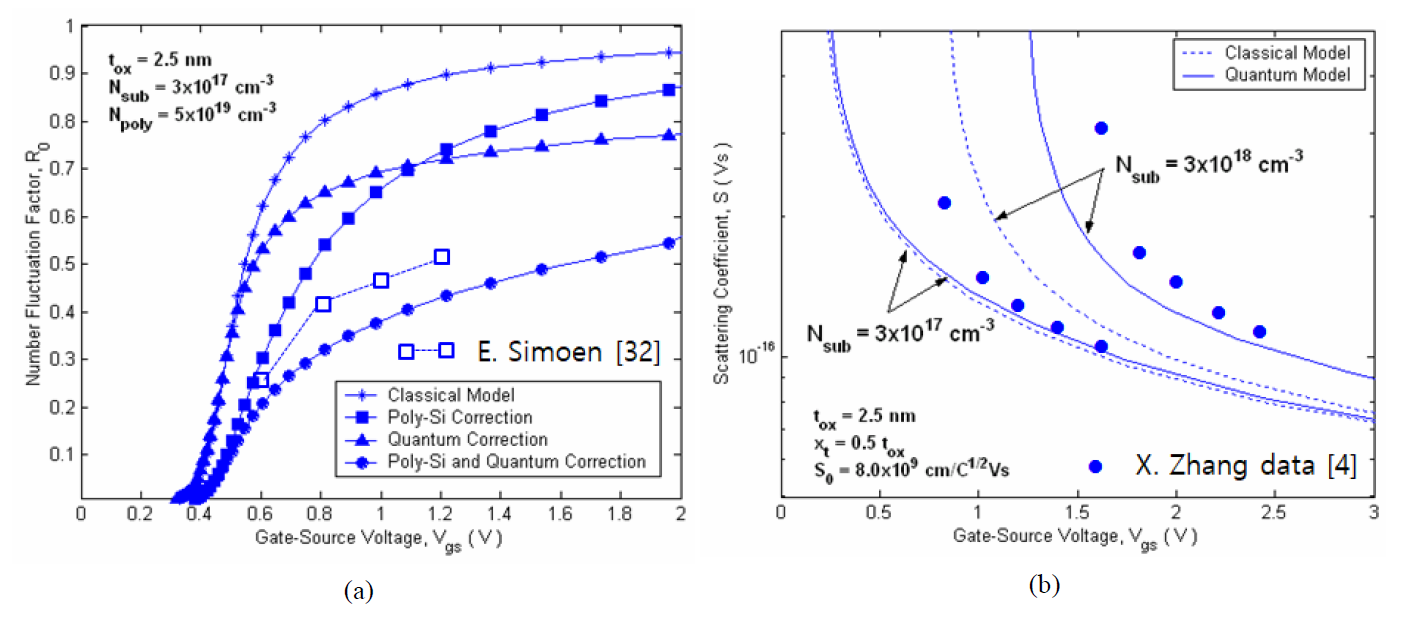Fig. 4. Quantum effects on the fractional RTS amplitude$\Delta I_{d} / I_{d}$versus the surface potential s y ($t_{ox}$= 2.5 nm,$N_{sub}$=3×1017 cm-3,$V_{FB}$= -0.9 V,$E_{ij}$=$E_{10}$,$S_{0}$= 8×109 cm/C1/2Vs,$k_{bulk}$= 0.625,$T$= 300 K).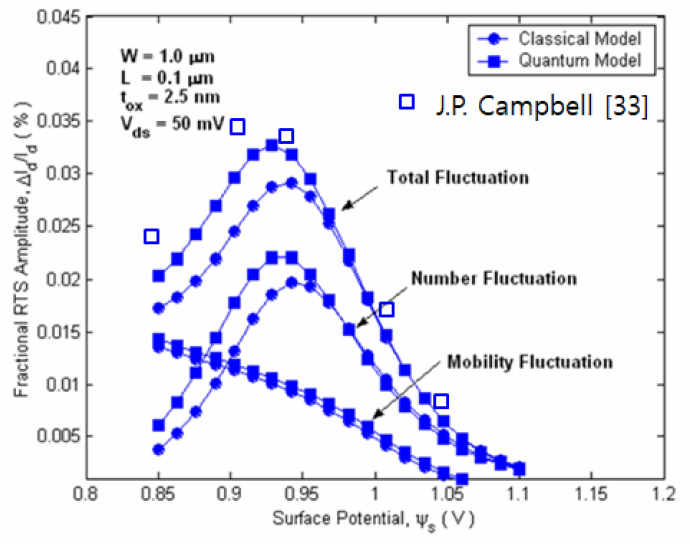Fig. 5 shows the comparison of measured data for$1/f$drain current noise and simulation results carried out with the classical noise model, accurately predicting the noise behavior. However, for higher doping concentration and shorter channel length, the deviations are pronounced, as illustrated in Fig. 6(a). The classical and QM models of$1/f$drain current noise behave in a qualitatively similar fashion to the RTS noise in the weak and strong inversion, showing the deviation in the saturation region. It can be seen for highly scaled devices ($L=0.1 \mu \mathrm{m}$) that QM model reproduces measurements in  relatively good for all bias conditions, whereas classical model has specific insufficiencies, especially for higer darin-source voltage. Fig. 6(b) illustrates the thermal noise spectral power density of drain current as a function of the drain-source voltage for different gatesource voltage. The simulation is verified with data extracted from noise measurements. The inclusion of QM effects can improve the accuracy of the thermal noise model in nanoscale channel length. Fig. 5. Comparison of the simulation results of classical model and measured data of the drain current noise as a function of drain-source voltage for two channel lengths ($t_{ox}$= 2.5 nm,$N_{sub}$= 2×1016 cm-3,$N_{t}$=2×1017 cm-3eV-1).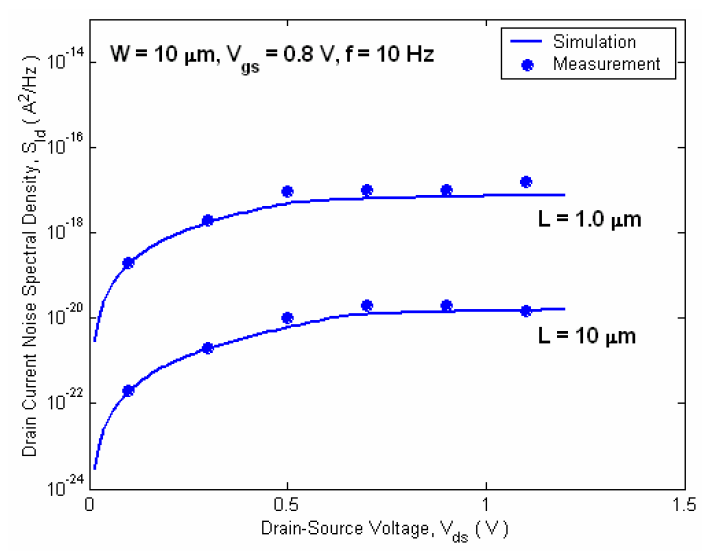Fig. 6. Quantum effects on (a) the$1/f$noise power of drain current versus the drain-source voltage$V_{ds}$, (b) the thermal noise power of drain current versus the drain-source voltage$V_{ds}$for two gate-source voltages ($N_{sub}$= 3×1017 cm-3,$V_{FB}$= -0.9 V,$E_{ij}$=$E_{10}$,$v_{sat}$= 8×106 cm/s,$R_{s,d}$=10 Ω,$k_{bulk}$= 0.625,$T$= 300 K).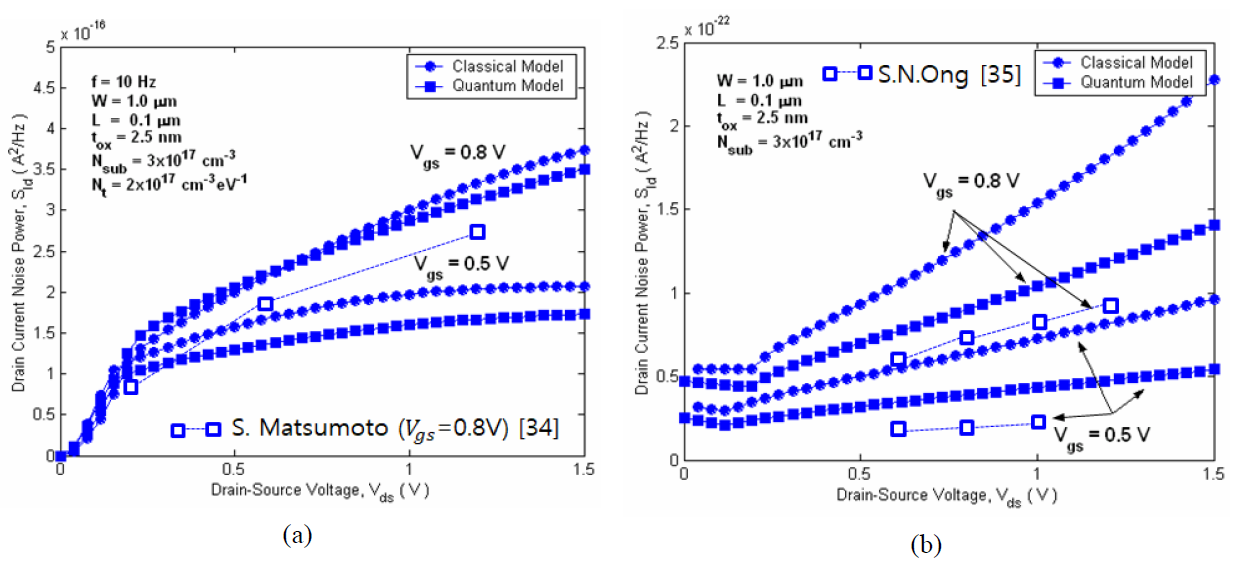The channel doping impacts are important to both the$1/f$noise and the thermal noise modeling. Obvious increases in the$1/f$noise are observed at higher doping concentration owing to channel non-uniformity, especially in sub-threshold region[36,37]. On the contrary, the thermal noise decreases for higher doping concentration, due to increased mobile charge. As shown in Fig. 7, it is confirmed that for higher doping concentration and thinner oxides, QM effects in the inversion layer should be considered for accurate noise modeling of nanoscale MOSFETs. Fig. 7. Quantum effects on the total noise power of drain current versus the frequency$f$for two substrate doping concentrations ($V_{FB}$= -0.9 V,$E_{ij}$=$E_{10}$,$v_{sat}$= 8×106 cm/s,$R_{s,d}$= 10 Ω,$k_{bulk}$= 0.625,$T\$ = 300 K).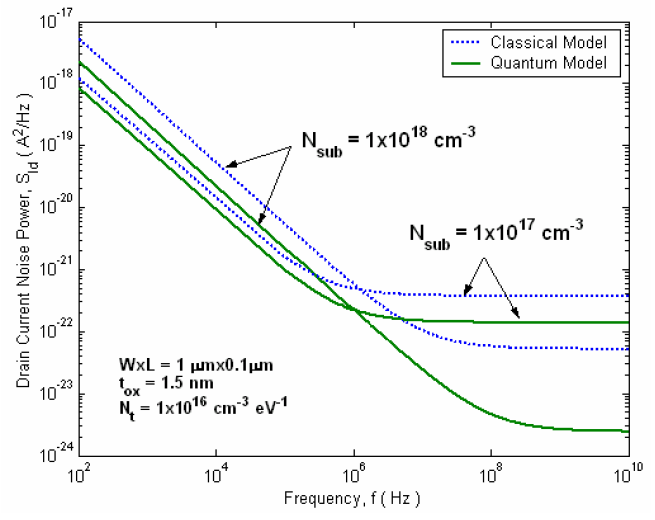## VI. CONCLUSION

The current and noise models accounting for QM effects in nanoscale MOSFETs were presented. Simple and analytical models were formulated by considering the QM effects due to the lowest energy level in the inversion layer. The inversion charge parameters were studied and treated quantum-mechanically, because good modeling of current and noise depends strongly on the accurate estimate of the inversion charge due to QM effects. The gate leakage current noise models were evaluated by using the quantum correction model for the oxide voltage and oxide thickness. By accounting for the QM effects through the inversion charge density model, the quantum models of the drain current and noise were obtained and compared with the classical models. The simulation results showed that 1) the quantum correction of the oxide thickness is essential to the accurate prediction of the gate leakage current and noise model, 2) QM effects on the 1/f noise and the thermal noise of drain current is significant in the saturation region, similar to the drain current characteristics, and 3) QM effects on the noise model become more pronounced for higher doing concentration and thinner oxide thickness. Therefore, as the MOS devices are scaled down to nanometer regime, existing current and noise models should incorporate more accurate, physics-based descriptions which account for quantization effects observable in the inversion layer.

### REFERENCES

1
Ando T., Fowler A. B., Stern F., 1982, Electronic properties of two-dimensional systems, Rev. Mod. Phys., Vol. 54, No. 2, pp. 437-6722
Huang J. Z., Chew W. C., Tang M., Jiang L., 2012, Efficient simulation and analysis of quantum ballistic transport in nanodevices with AWE, IEEE Trans. Electron Devices, Vol. 59, No. 2, pp. 932-9383
Karim M. A., Haque A., 2010, A physically based accurate model for quantum mechanical correction to the surface potential of nanoscale MOSFETs, IEEE Trans. Electron Devices, Vol. 57, No. 2, pp. 496-5024
Zhang X., White M. H., 2012, A quantum mechanical treatment of low frequency noise in high-K NMOS transistors with ultra-thin gate dielectrics, Solid-State Electron, Vol. 78, No. 12, pp. 131-1355
Moglestue C., 1986, Self-consistent calculation of electron and ole inversion charges at silicon-silicon dioxide interfaces, J. Appl. Phys., Vol. 59, No. 9, pp. 3175-31836
van Dort M. J., Woerlee P. H., Walker A. J., 1994, A simple model for quantization effects in heavily-doped silicon MOSFETs at inversion conductions, Solid-State Electron, Vol. 37, No. 3, pp. 411-4147
Liu W., Jin X., King Y., Hu C., 1999, An efficient and accurate compact model for thin-oxide-MOSFET intrinsic capacitance considering the finite charge layer thickness, IEEE Trans. Electron Devices, Vol. 46, No. 5, pp. 1070-10728
Larcher L., et al , 2001, A new model of gate capacitance as a simple tool to extract MOS parameters, IEEE Trans. Electron Devices, Vol. 48, No. 5, pp. 935-9459
Nakajima Y., et al , 1998, Physical origin and characteristics of gate capacitance in silicon metaloxide-semiconductor field-effect transistors, J. Appl. Phys., Vol. 83, No. 9, pp. 4788-479610
Janik T., Majkusiak B., 1994, Influence of carrier energy quantization on threshold voltage of metaloxide-semiconductor transistor, J. Appl. Phys., Vol. 75, No. 10, pp. 5186-519011
Ma Y., Li Z., Liu L., Tian L., Yu Z., 2000, Effective density-of-states approach to QM correction in MOS structure, Solid-State Electron, Vol. 33, No. 3, pp. 401-40712
Clerc R., et al , 2001, A physical compact model for direct tunneling from nMOS inversion layers, Solid-State Electron, Vol. 45, No. 10, pp. 1705-171613
Yang N., Henson W. K., Hauser J. R., Wortman J. J., 1999, Modeling study of ultrathin gate oxides using direct tunneling current and capacitancevoltage measurements in MOS devices, IEEE Trans. Electron Devices, Vol. 46, No. 7, pp. 1464-147114
Ip B. K., Brews J. R., 1998, Quantum effects upon drain current in a biased MOSFET, IEEE Trans. Electron Devices, Vol. 45, No. 10, pp. 2213-222115
Celik-Butler Z., Wang F., 2000, Effects of quantization on random telegraph signals observed in deep-sub micron MOSFETs, Microelectronics Reliability, Vol. 40, pp. 1823-183116
Lukyanchikova N. B., et al , 2000, Influence of the substrate voltage on the random telegraph signal parameters in submicron n-channel metal-oxidesemiconductor field-effect transistors under a constant inversion charge density, Appl. Phys. A, Vol. 70, pp. 345-35317
Pacelli A., et al , 1999, Quantum effects on the extraction of MOS oxide traps by 1/f noise measurements, IEEE Trans. Electron Devices, Vol. 46, No. 5, pp. 1029-103518
Palma A., et al , 1997, Quantum two-dimensional calculation of time constants of random telegraph signals in metal-oxide-semiconductor structure, Phys. Rev. B, Vol. 56, No. 15, pp. 9565-959419
Siergiej R. R., White M. M., Saks N. S., 1992, Theory and measurement of quantization effects in Si-SiO2 interface trap modeling, Solid-State Electron, Vol. 35, No. 6, pp. 843-85420
Hareland S. A., et al , 1996, A computationally efficient model for inversion layer quantization effects in deep sub-micron n-channel MOSFET’s, IEEE Trans. Electron Devices, Vol. 43, No. 1, pp. 90-9621
Lopez-Villanueva J. A., 1997, Effects of the inversion layer centroid on MOSFET behavior, IEEE Trans. Electron Devices, Vol. 44, No. 11, pp. 1915-192222
Ma Y., et al , 2001, Analytical charge-control and I-V model for sub-micrometer and deep-submicrometer MOSFETs fully comprising quantum mechanical effects, IEEE Trans. Computer-Aided Design, Vol. 20, No. 4, pp. 495-50223
Lee J. H., Bosman G., Green K. R., Ladwig D., 2002, Model and analysis of gate leakage current in ultrathin nitride oxide MOSFETs, IEEE Trans. Electron Devices, Vol. 49, No. 7, pp. 1232-124124
Lee J. H., Bosman G., Green K. R., Ladwig D., 2003, Noise model of gate leakage current in ultrathin oxide MOSFETs, IEEE Trans. Electron Devices, Vol. 50, No. 12, pp. 2499-250625
Masson P., et al , 1998, Influence of quadratic mobility degradation factor on low frequency noise in MOS transistors, Electronics Letters, Vol. 34, No. 20, pp. 1977-197926
Chow H. C., Feng W. S., 2003, An improved analytical model for short-channel MOSFETs, IEEE Trans. Electron Devices, Vol. 39, No. 11, pp. 2626-262927
Lee J. H., Bosman G., 2004, 1/fγ drain current noise model in ultrathin oxide MOSFETs, Fluctuation and Noise Letters, Vol. 4, No. 2, pp. L297-L30728
Jayaraman R., Sodini C. G., 1989, A 1/f noise technique to extract the oxide trap density near the conduction band edge of silicon, IEEE Trans. Electron Devices, Vol. 36, No. 9, pp. 1773-178229
Vandamme E. P., Vandamme L. K. J., 2000, Critical discussion on unified 1/f noise models for MOSFETs, IEEE Trans. Electron Devices, Vol. 47, No. 11, pp. 2146-215230
Wang B., Hellums J. R., Sodini C. G., 1994, MOSFET thermal noise modeling of analog integrated circuits, IEEE J. Solid-State Circuits, Vol. 29, No. 7, pp. 833-83531
Gaioni L., et al , Sep 2008, Instrumentation of gate current noise measurements on sub-100nm MOS transistors, Topical Workshop on Electronics for Particle Physics, pp. 436-44032
Simoen E., Mercha A., 2004, Experiemntal assessment of quantum effects in the low-frequency noise and RTS of deep submicron MOSFETs, in Advanced Experimental Methods for Noise Research in Nanoscale Electronic Devices. Kluwer Academic Publisher, pp. 121-12833
Campbell J. P., et al , May 2009, Large random telegraph noise in sub-threshold operation of nano-scale nMOSFETs, in Proc. IEEE Int. Conf. on IC Design and Technol, pp. 17-2034
Matsumoto S., et al , 2005, 1/f - noise characteristics in 100nm-MOSFETs and its modeling for circuit simulation, IEICE Trans. Electron, Vol. E88-C, No. 2, pp. 247-25435
Ong S. N., et al , 2009, A new unified model for channel thermal noise of deep sub-micron RFCMOS, IEEE Int. Symp. On Radio-Frequency Integration Technology, Singapore, pp. 280-28336
Chen J., Higashi Y., Hirano I., Mitani Y., Jun. 2013, Experimental study of channel doping concentration impacts on random telegraph signal noise and successful noise suppression by strain induced mobility enjancement, Proc. Symp. VLSI Technol.(VLSIT), pp. T184-T18537
Abe K., et al , 2010, Experimental investigation of effect of channel doping concentration on random telegraph signal noise, Jpn. J. Appl. Phys., Vol. 49, No. 4S, pp. 04DC07-138
Panda S., et al , 2013, Comparative study of thermal noise of Si surrounding gate MOSFET (SGMOSFET) with different gate oxides, Int. J. Semi. Sci. & Technol.(IJSST), Vol. 3, No. 2, pp. 17-22## Author

was born in Seoul, Korea, on February2, 1969.

He received the M.S. degrees from the Inha University, Incheon, Korea, and the Ph.D. degree in electrical & computer engineering from the University of Florida, Gainesville, in 1993 and 2003, respectively.

In 2017, he joined the Department of System Semiconductor Engineering, Sangmyung University, Korea, where he is currently a Professor.

From 2003 to 2016, he was with Samsung Display Inc., Korea, as a Principal Research Engineer, where he worked on semiconductor and display devices design and development.

His current research interests include semiconductor device modeling and simulation, physics-based artificial neural network, noise modeling in nanoscale MOSFETs, display device design and process-architecture, and thermoelectric transport modeling.

He has published more than 30 refereed journal and conference papers on these topics, and holds more than 20 patents in the field of display and semiconconductor devices.

was born in Seoul, Korea, in 1972.

He received the B.S. degree in Computer Engineering from Kwangwoon University, Seoul, Korea, in 1997, and the M.S. and Ph.D. degrees at Yonsei University, Seoul, Korea, in 1999 and 2003, respectively.

From 2002 to 2006, he was a Senior Researcher at the wireless PAN technology project office, Korea Electronics Technology Institute (KETI), KyungGi-Do, Korea.

In 2006, he joined the Department of System Semiconductor Engineering, Sangmyung University, Korea, where he is currently a Professor.

From 2016 to 2017, he served as dean of the Sangmyung University School of Engineering.

He is the leader of the PRIME, WeUp, and CK projects funded by national research foundation of Korea from 2014 to the present.

His research interests are in the areas of system on chip.